## Filtering Your Data using Python

Started ‎04-13-2016 by
Modified ‎04-16-2019 by
Views 727

# Filtering Your Data using Python

Sometimes you just want to look at a small subset of your data. Luckily, CAS makes this fairly easy to do through the use of WHERE clauses and variable lists. If you are using CASTable objects in Python, you can also use DataFrame-like operations on them to filter your view of the data. We'll look at some examples of both of these here.

The first thing we need to do is create a CAS connection.

``````In: import swat

Now we need some data to work with, so we'll upload the cars data set.

``````In: cars = conn.read_csv('https://raw.githubusercontent.com/sassoftware/sas-viya-programming/master/data/cars.csv')

In: cars

Using the len function in Python and the table.columinfo action, we can get some more information about the data in the CAS table. We see that it has 428 rows and 15 columns of data.

``````In: len(cars)
Out: 428

In: cars.table.columninfo()``````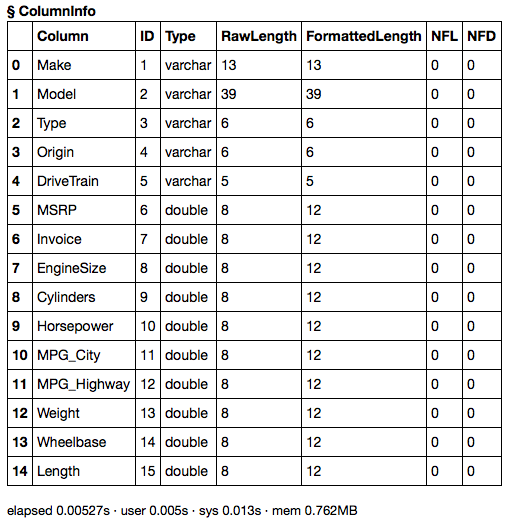Let's say that we only want to see the sports cars. We can do this in one of two ways. We could set the where parameter on the CASTable object to contain the string 'Type = "Sports"', or we could use the DataFrame data selection syntax. Let's look at the where parameter first.

To apply a WHERE clause to a CASTable, we can manually set the where attribute to the expression we want to apply.

``````In: cars.where = 'Type = "Sports"'

In: cars
Out: CASTable('_T_FBAD82B8_7F39899D30C0', caslib='CASUSERHDFS(kesmit)', where='Type = "Sports"')``````

We can look at the length and a sample of the data to see that it has been subset.

``````In: len(cars)
Out: 49Let's remove the where attribute and look at the DataFrame-like ways of subsetting data.

``````In: del cars.where

In: cars

The query method on the CASTable object mimics the query method of DataFrames. However, in this case, the syntax of the expression is the same as a CAS WHERE clause. So we use the same expression from above as the argument to the query method.

``In: cars.query('Type = "Sports"').head()``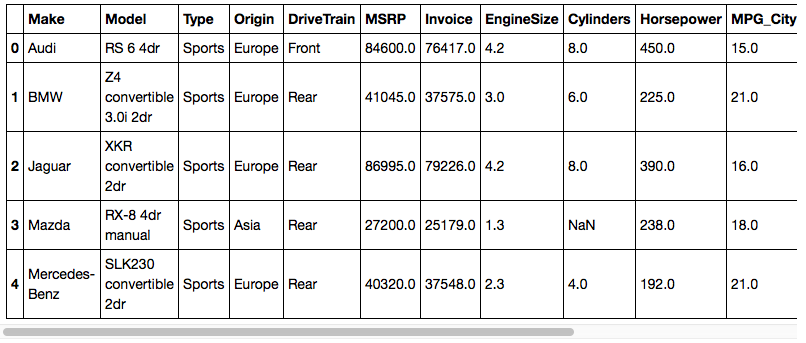Unlike setting the where parameter on the CASTable object, the query method does not embed the parameter in the CASTable object. It creates a copy of the table. If you want to apply the query to the CASTable object, you would add the inplace=True option.

``````In: cars.query('Type = "Sports"', inplace=True)

In: cars
Out: CASTable('_T_FBAD82B8_7F39899D30C0', caslib='CASUSERHDFS(kesmit)', where='Type = "Sports"')``````

A very popular way of subsetting the data in a DataFrame is using Python's getitem syntax (i.e., df[...]). You can use that same syntax on CASTable objects. First, we need to delete the WHERE clause that we had embedded using the last query method.

``````In: del cars.where

In: cars

The way to subset a table using DataFrame syntax is to index a CASTable object (e.g., cars[...]) using a condition on a column of that CASTable (e.g., cars.Type == 'Sports'). The condition is applied to the CASTable as a filter.

``In: cars[cars.Type == 'Sports'].head()``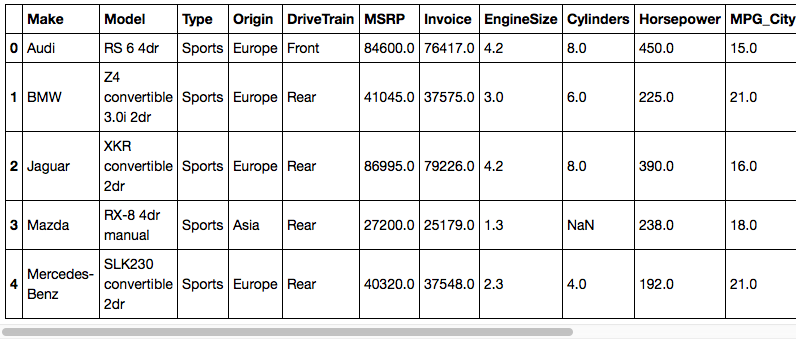The way this works is the condition creates a computed column that is treated as a mask. If you look at the result of the expression, you'll see that it creates a computed column describing the expression.

``````In: cars.Type == 'Sports'
Out: CASColumn('_T_FBAD82B8_7F39899D30C0', caslib='CASUSERHDFS(kesmit)', computedvars=['_eq_1_'], computedvarsprogram="_eq_1_ = (Type = 'Sports'); ")['_eq_1_']``````

If you look at a sample of the data created by the computed column, you'll see that it is a series of ones and zeros.

``````In: (cars.Type == 'Sports').head(40)
Out:
0     0.0
1     0.0
2     0.0
3     0.0
4     0.0
5     0.0
6     0.0
7     0.0
8     0.0
9     0.0
10    0.0
11    0.0
12    0.0
13    0.0
14    0.0
15    0.0
16    0.0
17    0.0
18    0.0
19    0.0
20    0.0
21    0.0
22    0.0
23    0.0
24    0.0
25    0.0
26    0.0
27    1.0
28    0.0
29    0.0
30    0.0
31    0.0
32    0.0
33    0.0
34    0.0
35    0.0
36    0.0
37    1.0
38    0.0
39    0.0
Name: _eq_J_, dtype: float64``````

When this mask is applied to a table, only the rows where the condition is true (i.e., computed expression is equal to one) show up in the output.

``In: cars[cars.Type == 'Sports'].head()``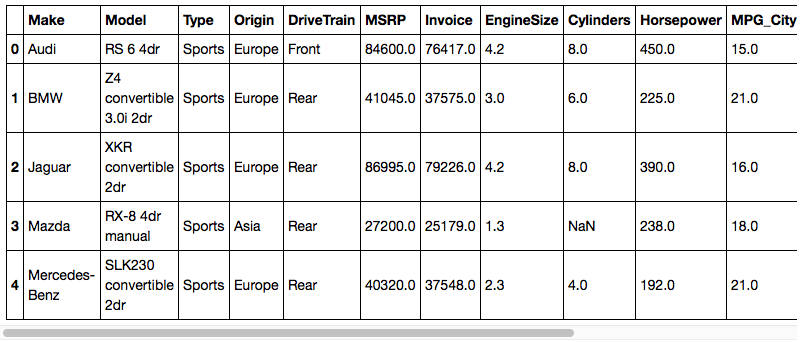It is also possible to combine expressions using Python's & and | operators. Due to the order of operations, you need to surround each subexpression with parentheses.

``In: cars[(cars.Type == 'Sports') & (cars.Cylinders > 6)].head()``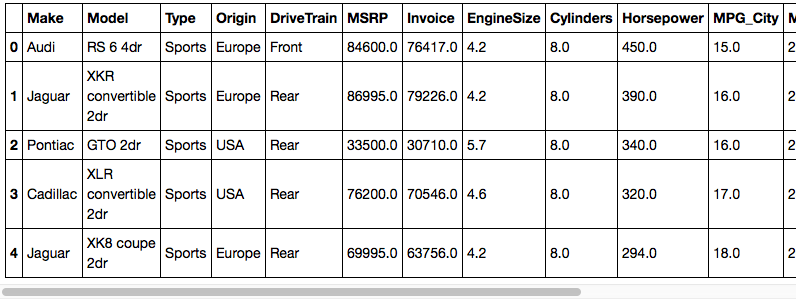Alternatively, you can chain your subsets which also results in combining those conditions into a single filter.

``In: cars[cars.Type == 'Sports'][cars.Cylinders > 6].head()``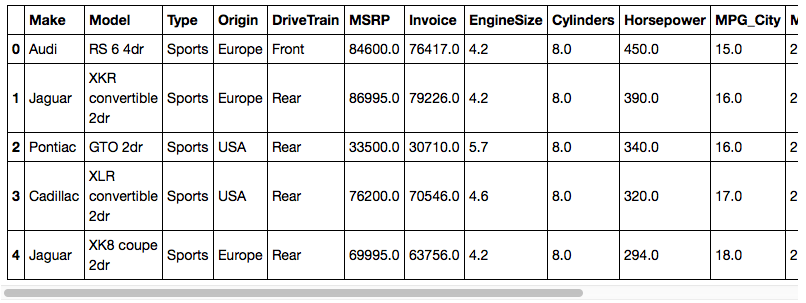If you want to see what's going on behind the scenes, you can always store the result in a variable. The resulting object is simply another CASTable object with a WHERE clause and computed columns for the expressions.

``````In: sports8 = cars[cars.Type == 'Sports'][cars.Cylinders > 6]

In: sports8
Out: CASTable('_T_FBAD82B8_7F39899D30C0', caslib='CASUSERHDFS(kesmit)', computedvars=['_eq_9_', '_gt_A_'], computedvarsprogram="_eq_9_ = (Type = 'Sports'); _gt_A_ = (Cylinders > 6); ", where='((_eq_9_)) and (_gt_A_)')[['Make', 'Model', 'Type', 'Origin', 'DriveTrain', 'MSRP', 'Invoice', 'EngineSize', 'Cylinders', 'Horsepower', 'MPG_City', 'MPG_Highway', 'Weight', 'Wheelbase', 'Length']]``````

``In: conn.close()``

## Conclusion

We've shown two different ways of filtering data in a CAS table. First we showed how you could manually apply a WHERE clause to a CASTable object. The next way of filtering data was based on the Pandas DataFrame API. You can either use the query method with a WHERE clause, or you can use the getitem syntax of Python to generate computed columns and WHERE clauses based on Python syntax.

## Resources